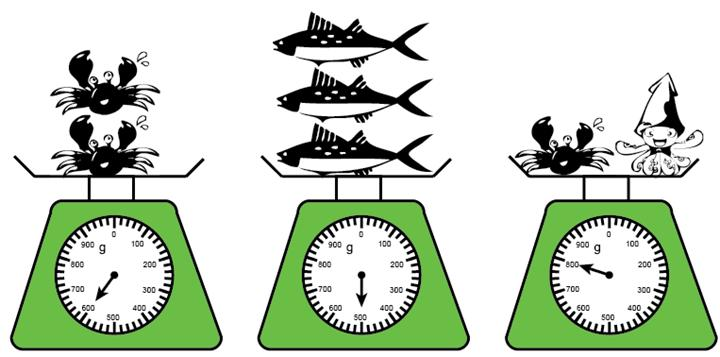### Sample Problem

Each crab weighs the same and each fish also weighs the same.(1) Each crab weighs g.

(2) The octopus weighs g.

(3) The octopus is g heavier than the three fishes.

(4) 1 crab is g lighter than 1 octopus.

(5) The total weight of the crabs and three fishes is g.

#### Solution

(1) The pointer is pointing to 600.

So the mass of each crab is 600 ÷ 2 = 300 g.

(2)  The pointer is pointing to 800.

So the mass of the octopus is 800 - 300 = 500 g.

(3)  The pointer is pointing to 500.

So the mass of the fishes is 500 g.

Difference between the octopus and the fishes: 500 - 500 = 0 g

(4) Difference between the crab and the octopus: 500 - 300 = 200 g

(5) Total weight of the crab and the three fishes: 600 + 500 = 1100 g